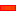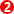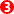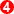﻿ Geared Five-Bar Kinematic-Chains

# Geared Five-Bar Kinematic-Chains

## Geared Five-Bar Mechanisms

Geared Five-bar mechanisms are built with:

 • One Gear-Pair

+

 • One Dyad - two Parts, three Joints.

####Geared-Rocker: Their Degrees-of-Freedom and Mobility

 Gruebler Equation: F = 3*(N–1) – 2*L- H F = 3 * (5–1) – 2*5 – 1 F = 12 – 10 – 1 F= 1 Degrees-of-Freedom = 1 Parts N = 5. Lower Pairs (Joints) L = 5 Higher Pairs (Cams or Gears) H = 1 There is one Gear-Pair A Geared Five-Bar mechanism has one Motion-Dimension that defines its Position. The Mobility - or Kutzbach Criterion - shows that a Motion-Part has a Mobility of Zero. Mobility =  Motion Dimensions – Degrees-of-Freedom Mobility = 1 – 1 = 0

#### Applications

Geared Five-bar mechanisms are often used:

 • To give complex Coupler Curves
 • As complex Function Generators

The Coupler Curves and the Functions can be more complex than available from Four-bar mechanisms.

#### Geared Five-bar Mechanism Configurations

When you assemble a Geared Five-bar Mechanism, you can edit the:

 1 Gear-Pair: - Fixed Gear Centre or Orbiting Gear Centre
 2 Dyad: - R-R-R, R-R-P, RPR, RPP, or PRP
 3 Gear Mesh - External Mesh or Internal Mesh
 4 Ratio of Gear-Teeth
 5 Lengths of the Part

### Typical Geared Five-bar Mechanism Configuration

####Complex Function Generators: Geared Five-bars with Pin-Joints only.In a Geared Five-bar, three Parts are the

 • Base-Part, Input Crank, and Geared-Rocker

The other two Parts are joined as a Dyad. Frequently, the Dyad is an R-R-R Dyad.

 STEP 1: Add a Simple Gear-Pair - Option 1

Step 1 is complete.STEP 2: Add an R-R-R Dyad

To remind you:

 2.a. Add two Parts

Step 2.a is complete.2.b. Add three Jointsbetween the Parts that are the Gear Pairs.

Step 2.b is complete.

Geared Five-Bar Mechanisms can give unusual motions and complex coupler curves.You may want to be more flexible with the design

 STEP 3: Edit the Part used for Gear 2

As an alternative to the R (Pin-Joint) at the end of the Part used for Gear 2, add a Point (with a Line) in the Part.

Use the new Point for one of the Pin-Joints in the R-R-R Dyad. You can edit the phase of the Gear 2 relative to Gear 1.

The design parameter options are:

 1 Gear Ratio between Gear 1 and 2 (Number-of-Teeth), Module to give centre distance.
 2 Phase between the Gears
 3 Length of Gear 'Cranks'
 4 Length of Dyad Parts
 5 Position of Coupler PointYou can change the gear ratio to give more complex coupler curves.

 STEP 4: Change the number-of-teeth with the Gear-Pair dialog-box - for example 60:40.

In this case, it takes two rotations of the input crank to complete the function at the output shaft

To plot the complete Trace-Point ,you must rotate the input crank two times faster.

 STEP 5: Add a Gearing FB; make the Gear ratio 2
 STEP 6: Connect the wire between the Linear-Motion FB, Gearing FB and the Motion-Dimension FB
 STEP 7: Connect the Output from the Motion-Dimension FB to the X input of the Graph FBHere is an interesting Coupler Curve.

In these Coupler Curves we are plotting the Point of the Pin-Joint.

You can add a Point to one of the Parts to give even more complex Coupler Curves.

####Complex Function Generators: Geared Five-bars with Pin-Joints only.Typically, you can get interesting output motions from a Geared Five-bar that has a Gear-Pair with an Orbiting Centre.

The output motion is a function of the input constant speed motion and is therefore called a Function-Generator.

 STEP 1: Add an Epicyclic Gear-PairSTEP 2: Make the gear ratio 1:1 (for example 50:50 Gear Teeth)

Step 2 is complete.STEP 3: Add an R-R-R Dyadbetween the end the Geared Rocker and the Line in the Base-Part

Step 3 is complete.STEP 4: Measure the angular position of the output Part over a Machine Cycle with a Measurement FBSTEP 5: Add a Graph FBSTEP 6: Connect the Measurement FB to an input of the Graph FB

Step 6 is complete.

Add a Design-Set to give a quick way to edit the Part lengths.This Graph shows the Output Shaft Rotation as a Function of the Input, Constant Speed, Shaft Rotation.

Notes about Mechanism Synthesis

Typically, the output motion is given as a function of the input. Then a mechanism is found that has an output Part that moves with the necessary function when the input Part moves.

Four-bar mechanism Function-Generators are limited. For example, it is not easy to synthesise a mechanism that oscillates the output shaft more than one time in a machine cycle.

It is clear from this graph that more complex functions are possible with Geared Five-bar mechanisms.Change the Gear Ratio to give more interesting Function Generation

You can change the gear ratio of the Gear-Pair to give more complex function generation.

 STEP 7: Change the Gear ratio - for example 60:40.

In this case, it takes two rotations of the input crank to complete the function at the output shaft

 STEP 4: Add a Gearing FB; make the Gear ratio 2
 STEP 5: Connect the Linear-Motion, Gearing and the Motion-Dimension FBs
 STEP 6: Connect the Output from the Motion-Dimension FB to the X input of the Graph FBThe Graph will show the Y-axis for two rotations of the crank to give the complete Function-Generation for the 60:40 gearing ratio.

####Complex Function Generators: Geared Five-bars with Pin-Joints and Slide-JointsGear-Pair, 1:1, Fixed-Centres, RPR Dyad.

Application: Coupler CurveGear-Pair, 2:1 Fixed-Centres with an RPR Dyad

Application: Coupler Curve

 • The Gear-Pair ratio changed to 60:40
 • The Crank must rotate twice for the mechanism to repeat a machine cycle.
 • To plot the complete Coupler Curve you should put in a Gearing FB before the Motion-Dimension FB and make the Gear Ratio 2.Gear-Pair, Fixed-Centres, R-R-P Dyad.

Application: Coupler CurveGear-Pair, Orbiting-Centre, RPR Dyad

Application: Function-GenerationThe 'Function' at the output Rocker.

It has a reasonable dwell.

Tutorials and Reference Help Files for MechDesigner and MotionDesigner 14.2 + © Machine, Cam, Mechanism, and Motion Design Software by PSMotion Ltd